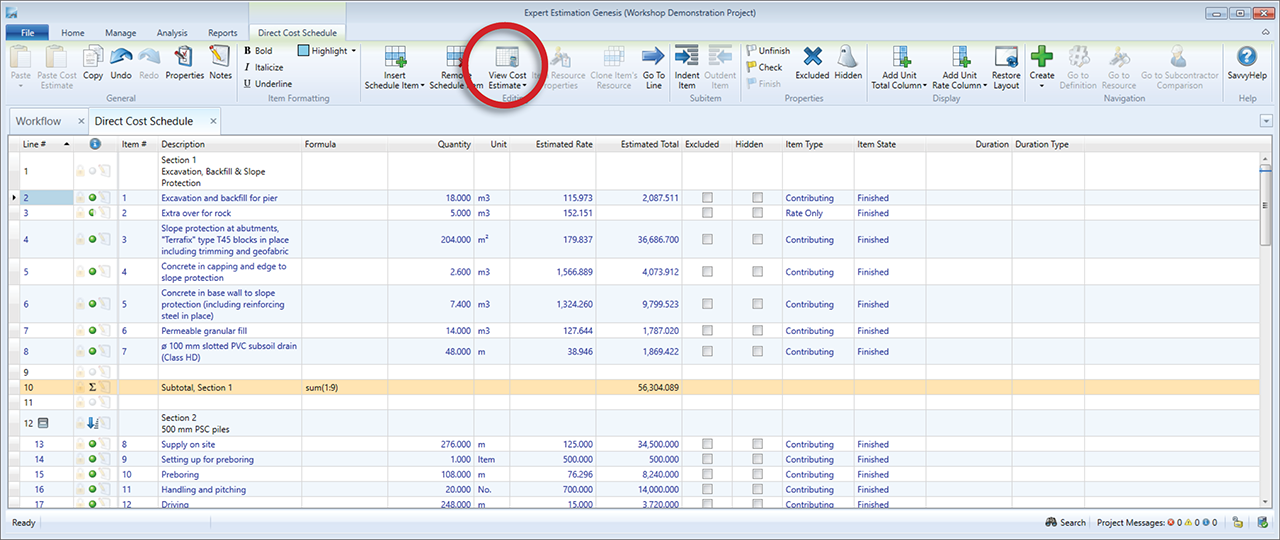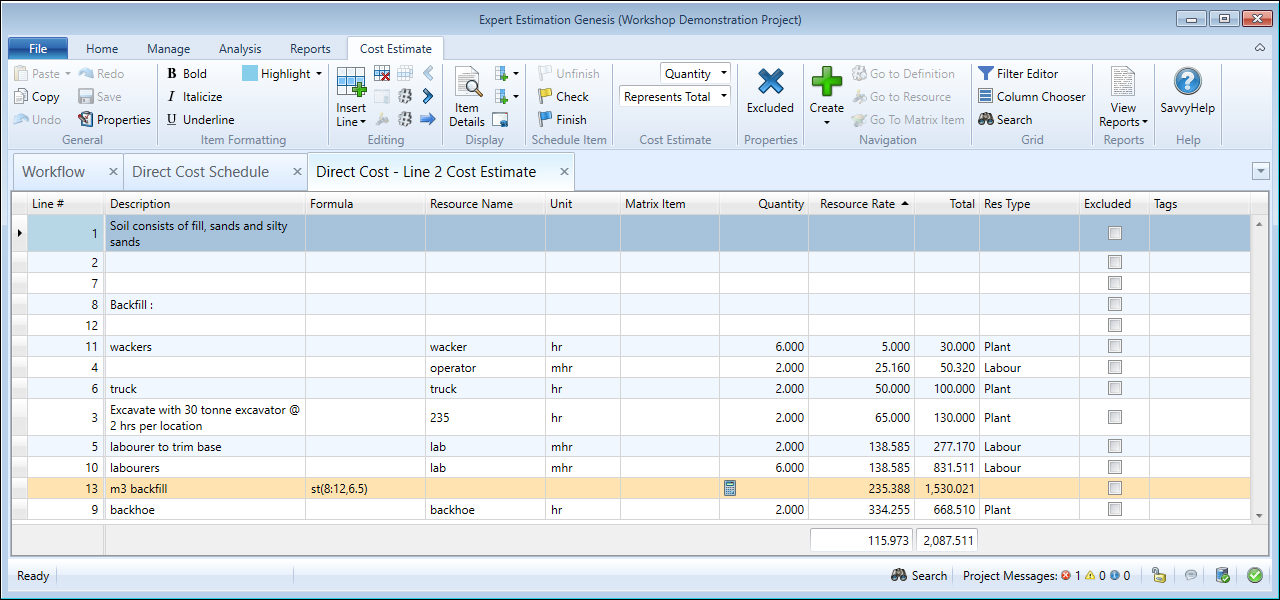## Cost Estimate

Cost Estimates allow you to build up the costs associated with a Schedule Item, Matrix Item or Group Resource using Resources and a wide variety of functions to generate value.

Cost Estimates are available in various locations throughout Expert Estimation and can be accessed by clicking on the View Cost Estimate button from the ribbon menu.Cost Schedule Window with the View Cost Estimate button highlighted

Cost Estimates are used in:

Each line is used to represent the individual resources required to complete a section of work, each creating a Total. The total value of the Cost Estimate is the sum of each contributing Cost Estimate Line Total. When used on a Schedule, a Cost Estimate Total can represent either the Rate or Total by setting the corresponding Cost Estimate Represents Mode.Example of the Cost Estimate Window

The contextual ribbon menu at the top of the window shows all the functions available within the Cost Estimate. The main grid area is where the Cost Estimate lines are displayed when using a Cost Estimate.

Within the Cost Estimate window, you can perform a variety of tasks that will build the costs of a section of work, along with a number of other functions such as: